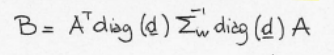# One or more output arguments not assigned

Hi all, I want to implement a convex-relaxation for the E-optimality design criterion, that is:
By definingthe optimization problem would be

I have implemented in Matlab using this function

``````function [d] = e_optimality(Uk, s, sigma)
%% E-OPTIMALITY DESIGN CRITERION, CONVEX RELAXATION
%
%       OUTPUT: sampling_set= vector with ordered sampling
%       set
%
%       INPUT: Uk= NxK Laplacian eigenvector in the selected band
%              s= number of samples
%              sigma: entry noise matrix

N= size(Uk,1);
Sigma= diag(sigma*ones(N,1))
Sigma_inv= inv(Sigma);

cvx_begin
variable d(N)
D= diag(d)
B= Uk'*Sigma_inv* D*Uk;
minimize -lambda_min(B)
subject to
d*ones(N,1)==s;
0<=d<=1;
cvx_end
``````

Unfortunately, when launghing the function I have the following error:

``````One or more output arguments not assigned during call to "varargout".

Error in minimize (line 14)
x = evalin( 'caller', sprintf( '%s ', varargin{:} ) );

Error in e_optimality (line 20)
minimize -lambda_min(B)
``````

It seems to be the minimize -lambda_min in CVX causing the problem. What could it be?
Thank you

Did you actually look at what CVX produced?

CVX accepted my `minimize -lambda_min(B)` statement.

`d*ones(N,1)==s`
is erroneous because `variable d(N)` makes `d` an `N by 1` vector (not 1 by N), so it is not conformal with `ones(N,1)`. So either change it to `d'*ones(N,1)` or `ones(1,N)*d` or `variable d(1,N)` or, more simply. if you can get into the MATLAB mentality and away from the Boyd and Vandenberghe book convention, just use `sum(d) == s`.
Please show the complete output from `cvx_version` , provide a complete reproducible example complete with all input data, as well as all CVX output. Please make the example as small in size as possible, and simplify, if possible, such as not wrapping the CVX code in a MATLAB function, provided that the simplified version still exhibits the error.